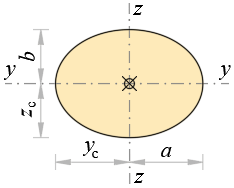### Geometrical Properties of EllipseDimensions

a = m, b = m

Area

A = π·a·b m2

Centroid

yc = a m, zc = b m

Perimeter (approx.)

P = π·(3·(a + b) –  (3·a + b)·(a + 3·b)) m

Second moments of area

Iy = π·a·b34 m4

Iz = π·b·a34 m4

Polar moment of area

Ix = Iy + Iz m4

Torsional constant

It = π·a3·b3a2 + b2 m4

Torsional section modulus

#if

a > b

Wt = π·a·b22 m3

#else

a < b

Wt = π·b·a22 m3

#end if